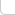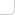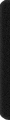# 某島

… : "…アッカリ～ン . .. . " .. .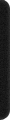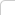June 23, 2012

## Codeforces Round #125

### Brief description:

Problem A. About Bacteria
… 培養皿中的 x 只細菌下一秒會繁衍出 kx+b 只細菌。。（。。。

。。。z 為初始為一隻細菌時，繁衍 n 秒的細菌總數。。。（。。。
(略，算術題。。不要把 z 真給算出來即可。。

Problem B. Jumping on Walls

。。問最終能出跳出這個坑。。
（略， Hash Dp 或者 BFS() 都行。。。。

Problem C. Delivering Carcinogen

。。飛船運行過程中距恆星的距離不得低於 r。。(r ≤ R) …

Problem D. Cube Snake

### Analysis:

Problem C. Delivering Carcinogen

。。。先旋轉 s 點到 x 軸上。。。考慮邊界情況、把 p 點移動到距離 s 點最遠的仍可直線訪問的位置。。（稱為臨界情況。

。。（同時注意到 2條切線 + 1段圓弧情況時 2條切線 的長度固定不變，就是圖中的 pm + ms 。

（代碼中的 alpha 是初始旋轉角，beta 是臨界情況時的 ∠sOp 。。
。。 theta 是當前 p 點具體所在位置。。。delta 是扇形的弧度。。）

const int N = 100009;

Po s, p, o;
DB R_, R, r, ss, vs, vp;
DB alpha, beta, theta, delta;

inline void _R(DB &x){
x = fmod(x, 2*PI); if (x < 0) x += 2*PI;
}

DB d(){
if(theta <= beta || theta >= 2*PI - beta) return sqrt(sqr(R_) + sqr(R) - 2*R_*R*cos(theta));
else{
delta = PI - beta - fabs(PI - theta);
return ss + delta * r;
}
}

bool f(DB x){
//cout << x << endl;
theta = x * vp + alpha, _R(theta);
return d() <= vs * x;
}

int main(){

#ifndef ONLINE_JUDGE
freopen("in.txt", "r", stdin);
#endif

p.input(), RD(vp), s.input(), RD(vs, r);

R_ = s.length(), R = p.length(), vp /= R;
ss = sqrt(sqr(R_) - sqr(r)) + sqrt(sqr(R) - sqr(r));
alpha = p.atan() - s.atan(), _R(alpha);
beta = acos(r / R_) + acos(r / R), _R(beta);

DB ll = 0, rr = (ss + PI*R) * vs;
REP(i, 100){
DB m = (ll + rr) / 2;
if (f(m)) rr = m; else ll = m;
}
OT(ll);
}


Problem D. Cube Snake

### Code: